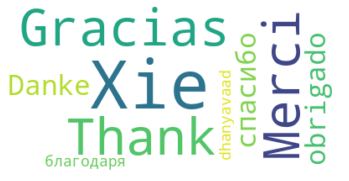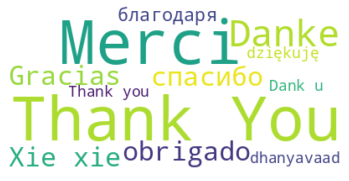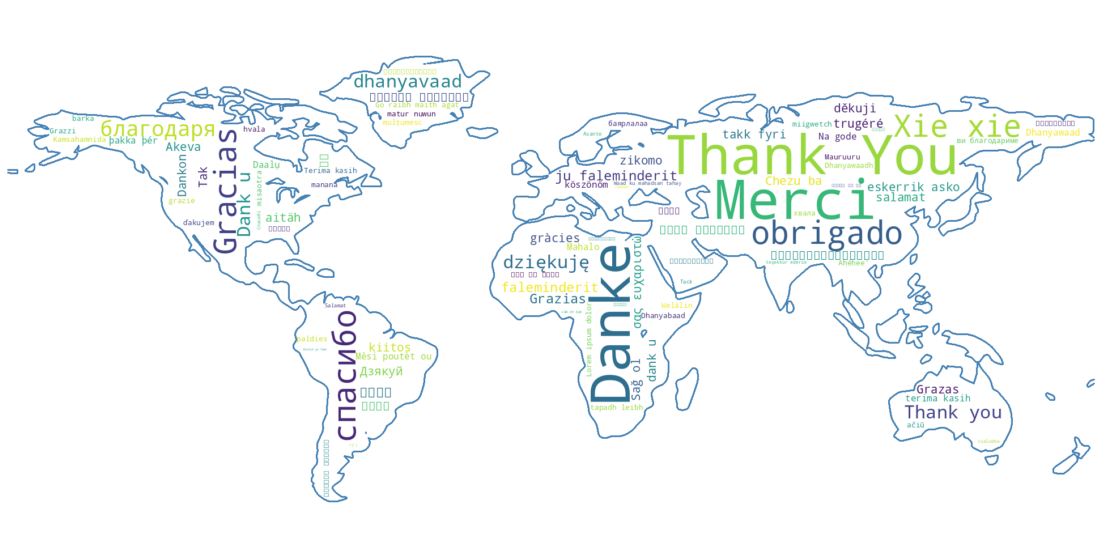To create Word Cloud in Python we will use a library called wordcloud.

It can be installed by:

``````pip install wordcloud
``````

Word cloud also known as:

• tag cloud
• wordle
• weighted list

is a visual representation of text data.

## Step 1: Create word cloud from text

For the first example we will create word cloud from a string.

If the word wight doesn't matter we can use method - `wc.generate(text)`:

``````from wordcloud import WordCloud
import matplotlib.pyplot as plt

wc = WordCloud(background_color="white", random_state=None)

text = " Thank You Gracias Merci Danke obrigado спасибо Xie xie благодаря dhanyavaad"

wc.generate(text)
plt.imshow(wc, interpolation="bilinear")
plt.axis('off')
plt.show()
``````## Step 2: Create word cloud by frequency

If the word order matters then we can use the method: `wc.generate_from_frequencies(freq)`.

This method requires dictionary of words with their frequency:

``````freq = {
"Thank You": 100,
"Merci": 99,
"Danke": 98,
``````

Below you can find full example of generating word cloud with word frequency:

``````from wordcloud import WordCloud
import matplotlib.pyplot as plt

wc = WordCloud(background_color="white")
freq = {
"Thank You": 100,
"Merci": 99,
"Danke": 98,
"спасибо": 96,
"Xie xie": 95,
"Gracias": 94,
"благодаря": 93,
"dziękuję": 91,
"Thank you": 90,
"Dank u": 89
}

wc.generate_from_frequencies(freq)

plt.imshow(wc, interpolation="bilinear")
plt.axis('off')
plt.show()
``````

the result is:## Step 3: Create word cloud from shape

Finally let's create a word cloud masked with a shape.

We are going to generate a word cloud from 100 ways to say "Thank you!" in 100 different languages. The code is below:

``````from wordcloud import WordCloud
import matplotlib.pyplot as plt
from PIL import Image
import numpy as np

freq = {
"Thank You": 100,
"Merci": 99,
"Danke": 98,
"спасибо": 96,
"благодаря": 95,
"o ṣeun": 1,
"Ngiyabonga": 0**
}

wc.generate_from_frequencies(freq)
plt.figure(figsize=(20,10) )
plt.imshow(wc, interpolation="bilinear")
plt.axis('off')
plt.show()
``````

Below you can find the result of the code above:Few things to have in mind when you are working with mask:

• the image used for a mask - should have white background. If you try to use others - it will break the shape.
• Free shapes can be taken from: pixabay - just edit and add background
• Sometimes the size of the output image might not respect the parameters passed to `WordCloud()`